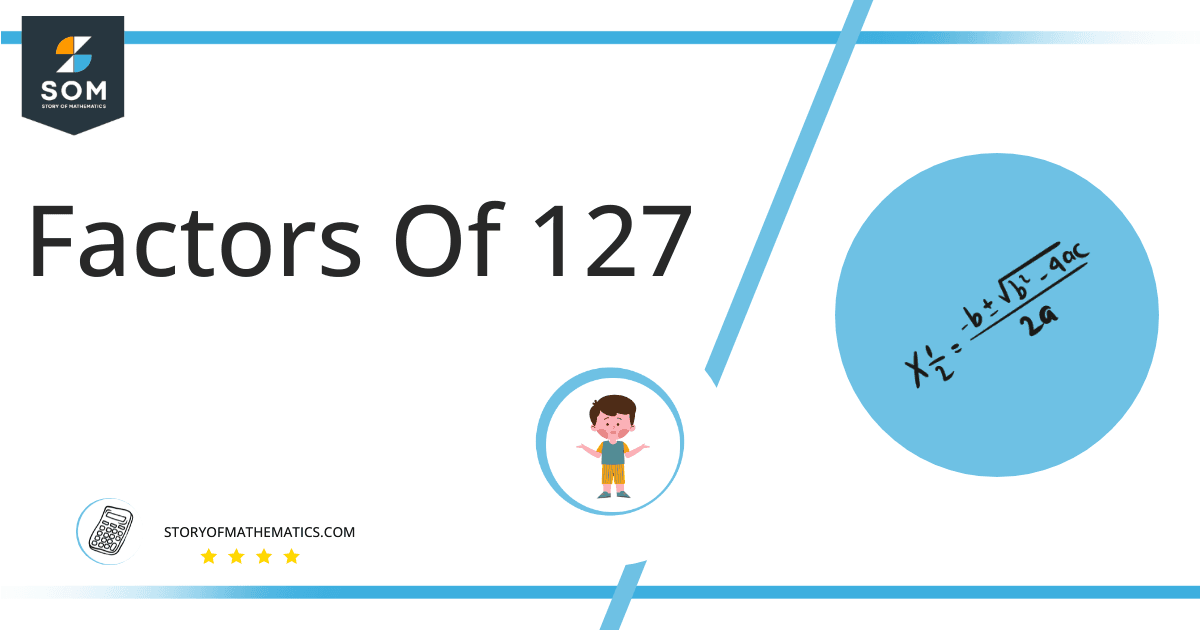# Factors of 127: Prime Factorization, Methods, and Examples

The natural numbers that completely divide 127 with zero remainders are called the factors of 127. 127 itself is a prime number which indicates that it cannot be factored into any other numbers except for 1 and 127 itself.### Factors of 127

Here are the factors of number 127.

Factors of 127: 1 and 127

### Negative Factors of 127

The negative factors of 127 are similar to its positive factors, just with a negative sign.

Negative Factors of 127: -1 and -127

### Prime Factorization of 127

The prime factorization of 127 is the way of expressing its prime factors in the product form.

Prime Factorization: 1 x 127

In this article, we will learn about the factors of 127 and how to find them using various techniques such as upside-down division, prime factorization, and factor tree.

## What Are the Factors of 127?

The factors of 127 are 1 and 127. All of these numbers are the factors as they do not leave any remainder when divided by 127.

The prime factors of the number 127 can be determined using the prime factorization technique.

## How To Find the Factors of 127?

You can find the factors of 127 by using the rules of divisibility. The divisibility rule states that any number, when divided by any other natural number, is said to be divisible by the number if the quotient is the whole number and the resulting remainder is zero.

To find the factors of 127, create a list containing the numbers that are exactly divisible by 127 with zero remainders. One important thing to note is that 1 and 127 are 127’s factors as every natural number has 1 and the number itself as its factor.

1 is also called the universal factor of every number. The factors of 127 are determined as follows:

$\dfrac{127}{1} = 127$

$\dfrac{127}{127} = 1$

Therefore, 1 and 127 are the only factors of 127.

### Total Number of Factors of 127

For 127 there are 2 positive factors and 2 negative ones. So in total, there are 4 factors of 127.

To find the total number of factors of the given number, follow the procedure mentioned below:

1. Find the factorization/prime factorization of the given number.
2. Demonstrate the prime factorization of the number in the form of exponent form.
3. Add 1 to each of the exponents of the prime factor.
4. Now, multiply the resulting exponents together. This obtained product is equivalent to the total number of factors of the given number.

By following this procedure the total number of factors of 127 is given as:

The factorization of 127 is 1 x 127.

The exponent of 1, 31, is 1 whereas that of 2 is 2.

Adding 1 to each and multiplying them together results in 4.

Therefore, the total number of factors of 127 is 12. 2 are positive and 2 factors are negative.

### Important Notes

Here are some important points that must be considered while finding the factors of any given number:

• The factor of any given number must be a whole number.
• The factors of the number cannot be in the form of decimals or fractions.
• Factors can be positive as well as negative.
• Negative factors are the additive inverse of the positive factors of a given number.
• The factor of a number cannot be greater than that number.
• Every even number has 2 as its prime factor which is the smallest prime factor.

## Factors of 127 by Prime Factorization

The number 127 is a prime number. Prime factorization is a useful technique for finding the number’s prime factors and expressing the number as the product of its prime factors.Before finding the factors of 127 using prime factorization, let us find out what prime factors are. Prime factors are the factors of any given number that are only divisible by 1 and themselves.

To start the prime factorization of 127, start dividing by its smallest prime factor. First, determine that the given number is either even or odd. If it is an even number, then 2 will be the smallest prime factor.

Continue splitting the quotient obtained until 1 is received as the quotient. The prime factorization of 127 can be expressed as:

127 = 1 x 127

## Factors of 127 in Pairs

The factor pairs are the duplet of numbers that when multiplied together result in the factorized number. Depending upon the total number of factors of the given numbers, factor pairs can be more than one.For 127, there is only one-factor pair as 127 is a prime number with only two factors.

The possible factor pair of 127 is given as (1, 127).

The negative factor pair of 127 is (-1, -127).

It is important to note that in negative factor pairs, the minus sign has been multiplied by the minus sign due to which the resulting product is the original positive number. Therefore, -1 and -127 are called negative factors of 127.

The list of all the factors of 127 including positive as well as negative numbers is given below.

Factor list of 127: 1, -1, 127, and -127

## Factors of 127 Solved Examples

To better understand the concept of factors, let’s solve some examples.

### Example 1

How many factors of 127 are there?

### Solution

The total number of Factors of 127 is 2.

Factors of 127 are 1 and 127.

### Example 2

Find the sum of factors of 127.

### Solution

The sum of factors of 127 is 1 + 127 = 128.

Therefore sum is equivalent to 128.